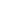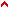1.2 Part 2 - extending the lines of the circle, square & triangle
In this part we propose to investigate the extension of circular lines, and we make this imaginary extension because a circular line, be it natural or artificially drawn with a compass, cannot be extended across a surface.
And now let us see how this investigation is done: in the first part, where we proved the quadrature and triangulature of the circle, we said that the capacity of the square is halfway between the capacity of the circle and that of the triangle; and thus we proved that line x.y., which is worth 4 units of the circle in the master figure, is worth half a unit more than the circular line of the circle, and that line a.b. is worth one half unit more than x.y.
Thus, we see that if the line of the circle in the plenary figure could be extended, it would only be worth three and a half units of line x.y. in which the square is extended, and line a.b. would be worth one unit of the square more than the circle, so that in the mind's eye, the extended circular line is half a unit shorter than the line of the square, and one unit shorter than the line of the triangle, while all the figures are equal in capacity.
Following what we said about the extended line of the whole circle which is worth 8 half-units of the square, we can discuss the parts of the lunules in the master figure, like circular line e. which has the same value as straight line ik, and as much more as the fourth part of 7 and a half units, or as a quarter unit of the straight line i.k.
We showed how circular lines are extended and how their extended value compares with that of straight lines, and following the example we gave with the master figure, examples can be given with figures of five, six or more angles, following the natural measurements of the capacity of the circle, square and triangle, and we just discussed this capacity here, in Part 2 of this book.
At this point we can consider how science originates in imaginary quantities drawn from quantities that are sensed, as when we make an imaginary measurement of a circular line by straightening it, and mentally measuring it with the straight lines of the square and the triangle, and these considerations expand the imagination's virtue as it feeds and verifies itself with imaginary species; in this way, one attains natural secrets inherent to the composition of figures, and we will give examples of this in the third part.Top